Next: MISSIONARIES AND CANNIBALS Up: CIRCUMSCRIPTION-A FORM OF NONMONOTONIC Previous: INTRODUCTION. THE QUALIFICATION PROBLEM

# THE NEED FOR NONMONOTONIC REASONING

We cannot get circumscriptive reasoning capability by adding sentences to an axiomatization or by adding an ordinary rule of inference to mathematical logic. This is because the well known systems of mathematical logic have the following monotonicity property. If a sentence q follows from a collection A of sentences and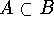, then q follows from B. In the notation of proof theory: if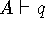and, then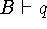. Indeed a proof from the premisses A is a sequence of sentences each of which is a either a premiss, an axiom or follows from a subset of the sentences occurring earlier in the proof by one of the rules of inference. Therefore, a proof from A can also serve as a proof from B. The semantic notion of entailment is also monotonic; we say that A entails q (written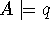) if q is true in all models of A. But ifand, then every model of B is also a model of A, which shows that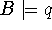.

Circumscription is a formalized rule of conjecture that can be used along with the rules of inference of first order logic. Predicate circumscription assumes that entities satisfy a given predicate only if they have to on the basis of a collection of facts. Domain circumscription conjectures that the ``known'' entities are all there are. It turns out that domain circumscription, previously called minimal inference, can be subsumed under predicate circumscription.

We will argue using examples that humans use such ``nonmonotonic'' reasoning and that it is required for intelligent behavior. The default case reasoning of many computer programs (Reiter 1980) and the use of THNOT in MICROPLANNER (Sussman, et. al. 1971) programs are also examples of nonmonotonic reasoning, but possibly of a different kind from those discussed in this paper. (Hewitt 1972) gives the basic ideas of the PLANNER approach.

The result of applying circumscription to a collection A of facts is a sentence schema that asserts that the only tuples satisfying a predicate P(x, ... ,z) are those whose doing so follows from the sentences of A. Since adding more sentences to A might make P applicable to more tuples, circumscription is not monotonic. Conclusions derived from circumscription are conjectures that A includes all the relevant facts and that the objects whose existence follows from A are all the relevant objects.

A heuristic program might use circumscription in various ways. Suppose it circumscribes some facts and makes a plan on the basis of the conclusions reached. It might immediately carry out the plan, or be more cautious and look for additional facts that might require modifying it.

Before introducing the formalism, we informally discuss a well known problem whose solution seems to involve such nonmonotonic reasoning.Next: MISSIONARIES AND CANNIBALS Up: CIRCUMSCRIPTION-A FORM OF NONMONOTONIC Previous: INTRODUCTION. THE QUALIFICATION PROBLEM

John McCarthy
Tue May 14 00:04:52 PDT 1996